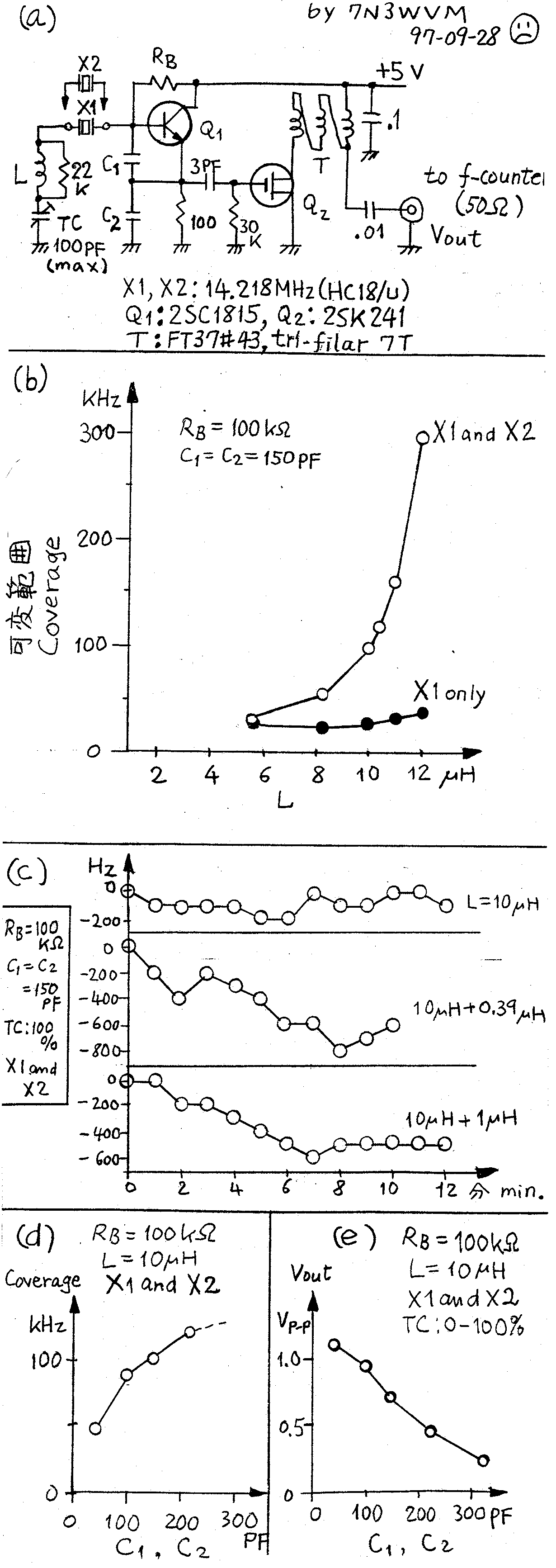Experiment on Super VXO
Cryptic letters are codes for Japanese/Chinese characters. English readers may skip them.

\$B?^(B(a)\$B\$,=H/?6;R\$G!"(BX1[email protected]\$1\$N>l9g!JIaDL\$N(BVXO\$B!K!"(BX1\$B\$H(BX2[email protected]\$b\$N(B \$B!J%9!<%Q!<(BVXO\$B!KN>J}\$N>l9g\$K\$D\$\$\$FB,Dj\$7\$^\$7\$?!#(BL\$B\$KJBNs\$KF~\$l\$F\$"\$k(B22\$B%-(B \$B%m%*!<%`\$NDq93\$O<~GH?t\$,[email protected]\$jMzNr8=>]\$,5/\$3\$i\$J\$\$\$h\$&\$K\$9\$k\$?\$a\$N\$b(B \$B\$N\$G\$9!#%P%\$%"%9Dq93(BRB\$B\$O(B100\$B%-(B \$B%m%*!<%`\$K\$7\$^\$7\$?!#\$3\$N;~(BQ1\$B\$N%(%_%C%?EEN.\$O(B9mA\$B\$G\$7\$?!#(BC1\$B\$H(BC2\$B\$O6&\$K(B 150pF\$B\$K\$7\$^\$7\$?!#(BRB\$B\$H(BC1\$B!"(BC2\$B\$K\$D\$\$\$F\$O.CM\$O(B [email protected]\$G\$9\$,\$*\$=\$i\$/?t(BpF\$B\$G\$7\$g\$&!#(B

\$B?^(B(b)\$B\$,(BL\$B\$rJQ\$(\$?;~\$N2DJQHO0O!J%H%j%^%3%s%G%s%5\$r:G>.\$H:GBg\$K\$7\$F(B \$B5a\$a\$k!KB,Dj\$N7k2L\$G\$9!#IaDL\$N(BVXO\$B\$H%9!<%Q!<(BVXO\$B\$N0c\$\$\$ONrA3\$H\$7\$F\$\$\$^\$9!#(B \$B%9!<%Q!<(BVXO\$B\$G\$O2DJQHO0O\$O2?\$H(B300kHz\$B\$K\$b5Z\$S\$^\$9!#(B \$B\$7\$+\$7!"NI\$\$\$3\$H\$P\$+\$j\$G\$O\$"\$j\$^\$;\$s!#(B

\$B\$\$\$m\$\$\$m\$J(BL\$B\$NCM\$N;~!"<~GH?t\$N;~4VJQ2=\$rD4\$Y\$?7k2L\$,?^(B(c)\$B\$K<(\$7\$F\$"\$j\$^\$9!#(B \$BH>ED\$E\$1\$N8e(B25\$BJ,BT\$C\$FEE8;\$rF~\$l\$FB,Dj\$r;O\$a\$^\$9!#(BL=10uH \$B\$O\$^\$"\$^\$"5v(B \$B\$;\$k\$b\$N\$N!"(BL=10.39uH\$B\$d(B11uH\$B\$N;~\$OJQF0\$,Bg\$-\$/\$^\$k\$G(BVFO\$B\$N\$h\$&\$G\$9!#(B \$B\$*\$=\$i\$/!"(BL[email protected]\$,1F6A\$7\$F\$\$\$k\$H;W\$\$\$^\$9!#0x\$_\$K!"\$3\$NFs\$D\$N(BL\$B\$N(B \$BCM\$K\$D\$\$\$F\$O\$3\$NCM\$N\$b\$N\$,\$J\$+\$C\$?\$N\$G!"?^(B(c)\$B\$K<(\$7\$?\$h\$&\$K\$U\$?\$D\$N(BL\$B\$r(B \$BD>[email protected]\B3\$7\$FMQ\$\$\$F\$\$\$^\$9!#(B \$B2DJQHO0O\$H0BDjEY\$r\$O\$+\$j\$K\$+\$1\$F(BL=10uH \$B\$"\$?\$j\$G2fK}\$9\$k\$N\$,\$\$\$\$(B \$B\$N\$G\$O\$J\$\$\$+\$H;W\$\$\$^\$9!#\$7\$+\$7\$b\$C\$HBg\$-\$J(BL\$B\$G\$b!"\$b\$&>/\$7BT\$F\$P0BDj\$9(B \$B\$k\$+\$bCN\$l\$^\$;\$s\$7!"[email protected]\$N>/\$J\$\$(BL\$B\$r;H\$&\$3\$H\$b9M\$(\$i\$l\$^\$9!#(B

\$B?^(B(d)\$B\$O!"(BC1\$B\$H(BC2\$B\$rF1;~\$K\$\$\$m\$\$\$mJQ\$(\$?;~\$K2DJQHO0O\$NJQ\$o\$kMM;R\$r<(\$7\$F(B \$B\$\$\$^\$9!#(BC1\$B\$H(BC2\$B\$rBg\$-\$/\$9\$k\$H2DJQHO0O\$bBg\$-\$/\$J\$j\$^\$9\$,!"?^(B(e)\$B\$K<(\$9\$h(B \$B\$&\$KH/?6=PNOEE05(BVout\$B\$b>.\$5\$/\$J\$j\$^\$9!#(B C1=C2=330pF\$B\$G\$O>.\$5\$/\$J\$j2a\$.\$F(B \$BB,Dj\$G\$-\$^\$;\$s\$G\$7\$?!#(B100\$B!A(B150pF\$B\$H\$\$\$&\$N\$ONI\$\$A*[email protected]\$H;W\$\$\$^\$9!#(B

\$B:G8e\$K!"%P%\$%"%9Dq93(B RB \$B\$rJQ\$(\$F\$_\$^\$7\$?!#Ev=i\$N(B100\$B%-%m%*!<%`\$+\$i(B 200 \$B%-%m%*!<%`\$K\$9\$k\$H%(%_%C%?EEN.\$O(B9mA\$B\$+\$i(B5.2mA\$B\$K8:>/\$7\$^\$7\$?\$,!"2DJQHO0O(B \$B\$K\$O1F6A\$"\$j\$^\$;\$s\$G\$7\$?!#C"\$7!"H/?6=PNOEE05(BVout\$B\$,(B0.7Vp-p\$B\$+\$i(B0.3Vp-p\$B\$K(B \$B8:>/\$7\$^\$7\$?!#(B RB \$B\$r\$5\$i\$KBg\$-\$/\$7\$F(B470\$B%-%m%*!<%`\$K\$7\$?\$i!"%(%_%C%?EEN.\$,(B2.4mA\$B\$K\$J\$j!"(B Vout\$B\$,>.\$5\$/\$J\$j2a\$.\$FB,DjITG=\$H\$J\$C\$F\$7\$^\$\$\$^\$7\$?!#(B

[email protected]\$H\$7\$F!"%9!<%Q!<(BVXO\$B\$G\$O(BL=10uH\$B\$G2DJQHO0OLs(B100 kHz \$B\$,[email protected]\$i\$l\$^\$7\$?!#(B \$B<~GH?t\$N0BDjEY\$O\$=\$3\$=\$3\$G\$9\$,!"(BL\$B\$rBg\$-\$/\$9\$k\$H0BDjEY\$O0-\$/\$J\$j\$^\$9!#(B C1\$B\$H(BC2\$B\$rBg\$-\$/\$9\$k\$H2DJQHO0O\$OBg\$-\$/\$J\$j\$^\$9!#\$7\$+\$7!"(BQ1\$B\$N%P%\$%"%9\$rJQ(B \$B\$(\$F\$b2DJQHO0O\$OJQ\$o\$j\$^\$;\$s\$G\$7\$?!#(B

\$BCm0U\$7\$J\$/\$F\$O\$J\$i\$J\$\$\$3\$H\$O!"[email protected]\$i\$l\$?7k2L\$O;H\$C\$??e>=H/?6;R\$KFC(B \$BM-\$N\$b\$N\$G\$"\$k\$3\$H\$G\$9!#0c\$&%Q%i%a!<%?\$N?e>=H/?6;R\$d0c\$&<~GH?t\$N?e>=H/(B \$B?6;R\$r;H\$(\$P!":GE,\$J(BL\$B\$d(BC1\$B!"(BC2\$B\$NCM\$b0[\$J\$C\$F\$-\$^\$9!#(B \$B=H/?6;R\$GAn experiment on VXO is done to investigate frequency coverage of an ordinary VXO and a super VXO. The super VXO was invented and named by JA0AS(I. Shimizu, Silent Key) and JH1FCZ(T. Okubo).

Figure(a) shows the schematic diagram of the experiment. X1 and X2 are HC18/U X'tals of nominal frequency 14.218 MHz. In the experiment, only X1 is connected in the place shown in the diagram for measurements of ordinary VXO. For measurements of super VXO, X2 is added in parallel to X1. Inductance L is the main parameter of this experiment. A 22-kilo ohm resistor is added in parallel to L in order to avoid a frequency skip or hysteresis. Q1 is a small signal general purpose transistor with fT = 80MHz. Q2 is a small signal cascode MOS FET with gm = 10 mS. The bias resistor RB was chosen to be 100 kilo ohms. With this value, emitter current of 9 mA flowed in Q1. C1 and C2 were both chosen to be 150pF. RB, C1 and C2 of different values were also tried at the end of this experiment. TC is a plastic trimmer capacitor with maximum capacitance of 100pF. Minimum capacitance is not known, supposedly several pF.

Results are shown in figure(b) on the frequency coverage as measured by varying TC from its minimum value to 100pF. The measurements were done with L of 5.6, 8.2, 10, 10.39, 11 and 12 uH. For 10.39uH, two inductors 10uH and 0.39uH are used in seires. For 11uH, 10uH and 1uH in series. A difference is prominent between ordinary VXO and super VXO. As L is increased, the coverage of ordinary VXO did not increase so much, while the coverage of super VXO increased rapidly up to about 300 kHz(!) with L=12uH. However, we have to be careful about that wide coverage.

See figure(c). Drift of the frequency in time is measured for L=10, 10.39, and 11uH. In this measurement, X1 and X2 are used in parallel, and TC is set at 100pF. A batch of measurements with a certain value of L was started 25 minutes after the soldering, and power supply was switched on just before the start of the batch. While the frequency with L=10uH is acceptably stable in time, the frequency is jumping or drifting considerably with L=10.39 and 11uH. The drift of the frequency is most probablly due to the temperature coefficient of the inductors, and due to the VFO-like nature of the oscillator. I would stop at L=10uH for the stable operation although you might eventually get better stability with larger L's if you wait longer time or if you use inductors with small temperature coefficients.

Now, I play a little with the capacitance of C1 and C2. All other parameters are fixed at RB=100kilo ohms, L=10uH, and X1, X2 in parallel. Figure (d) shows the frequency coverage as a function of capacitance of C1 and C2. C1 and C2 are varied simultaneously. You see that the coverage gets wider as the capacitance is increased. The measurement with C1=C2=330pF was not possible because Vout(output voltage to the frequency counter) got too small as is shown in figure(e). 100 to 150pF may be a good choice.

Finally, the bias resistor RB was varied. Other conditions, C1=C2=150pF, L=10uH, and X1, X2 in parallel were kept. Originally, RB was set at 100kilo ohms and emitter current was 9mA. This time, RB was cahnged to 200kilo ohms, then emitter current decreased to 5.2mA. The frequency coverage did not change although Vout decreased from 0.7Vp-p to 0.3Vp-p. RB was further increased to 470kilo ohms, then emitter current decreased to 2.4mA. However, Vout got too low, less than 0.1Vp-p with this low current. Therefore, frequency measurement was not possible.

In conclusion, frequency coverage of about 100 kHz was obtained with L=10uH, C1=C2=150pF, X1 and X2 put together in parallel and RB=100kilo ohms. The stability was acceptable, but got worse with larger L's. The coverage got wider as C1 and C2 were set at larger values but did not depend on the bias of Q1.

It should be emphasized that these results are more or less specific to the X'tals used in the present experiment. Optimum values for L, C1 and C2 may differ from the values obtained in this experiment if X'tals with different parameters or different frequencies are used. You need to do an experiment to optimize the parameters for your X'tals.

MINOWA, Makoto
7N3WVM
Last revised 1997-09-30.